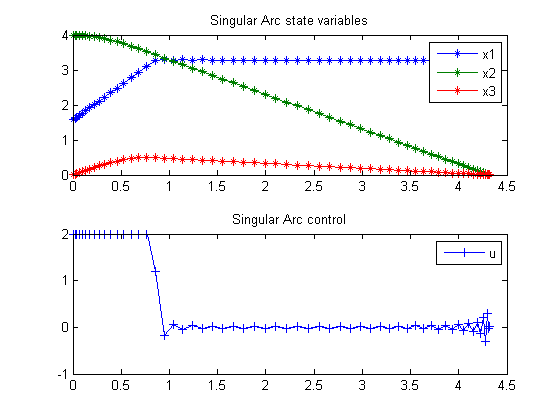99  Singular Arc Problem

Problem 3: Miser3 manual

99.1  Problem Formulation

Find u(t) over t in [0; t_f ] to minimize

 J = tf

subject to:

 dx1 dt
= u
 dx2 dt
= cos(x1
 dx3 dt
= sin(x1
 x2(tf) = x3(tf) = 0
 |u| <= 2
x(0) = [
 pi 2
4  0]

Reference: 

99.2  Problem setup

toms t
toms t_f
p = tomPhase('p', t, 0, t_f, 60);
setPhase(p);

tomStates x1 x2 x3
tomControls u

% Initial guess
x0 = {t_f == 20
icollocate({
x1 == pi/2+pi/2*t/t_f
x2 == 4-4*t/t_f; x3 == 0})
collocate(u == 0)};

% Box constraints
cbox = {2 <= t_f <= 1000
-2 <= collocate(u) <= 2};

% Boundary constraints
cbnd = {initial({x1 == pi/2; x2 == 4; x3 == 0})
final({x2 == 0; x3 == 0})};

% ODEs and path constraints
ceq = collocate({dot(x1) == u
dot(x2) == cos(x1); dot(x3) == sin(x1)});

% Objective
objective = t_f;

99.3  Solve the problem

options = struct;
options.name = 'Singular Arc';
solution = ezsolve(objective, {cbox, cbnd, ceq}, x0, options);
t  = subs(collocate(t),solution);
x1 = subs(collocate(x1),solution);
x2 = subs(collocate(x2),solution);
x3 = subs(collocate(x3),solution);
u  = subs(collocate(u),solution);
Problem type appears to be: lpcon
Starting numeric solver
===== * * * =================================================================== * * *
TOMLAB - Tomlab Optimization Inc. Development license  999001. Valid to 2011-02-05
=====================================================================================
Problem: ---  1: Singular Arc                   f_k       4.321198387073171600
sum(|constr|)      0.000000179336713690
f(x_k) + sum(|constr|)      4.321198566409885100
f(x_0)     20.000000000000000000

Solver: snopt.  EXIT=0.  INFORM=1.
SNOPT 7.2-5 NLP code
Optimality conditions satisfied

FuncEv    1 ConstrEv   77 ConJacEv   77 Iter   70 MinorIter  377
CPU time: 1.234375 sec. Elapsed time: 1.281000 sec.

99.4  Plot result

subplot(2,1,1)
plot(t,x1,'*-',t,x2,'*-',t,x3,'*-');
legend('x1','x2','x3');
title('Singular Arc state variables');

subplot(2,1,2)
plot(t,u,'+-');
legend('u');
title('Singular Arc control');